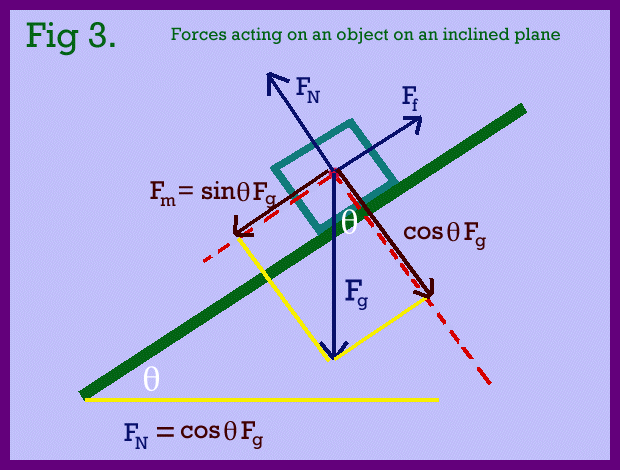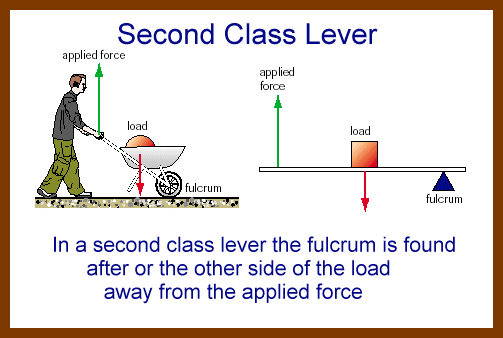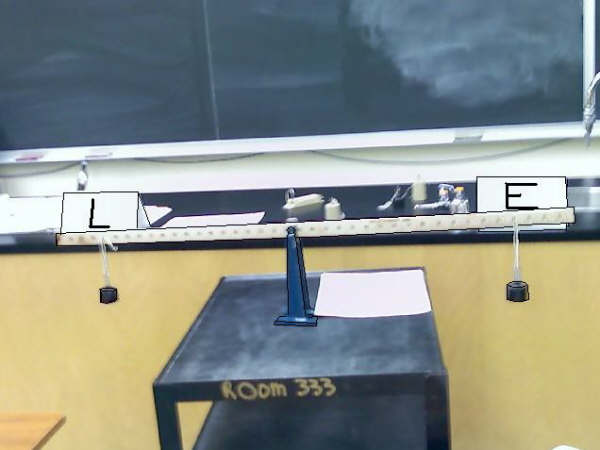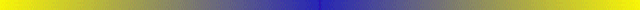# Mechanical Systems

## Mechanical systems is the study of forces and the application of forces

Mechanical Systems Overall Expectations
By the end of this course, students will:

• describe and apply concepts related to forces, Newton’s laws of motion, static and kinetic friction, simple machines, torques, and mechanical advantage;
• design and carry out experiments to investigate forces, coefficients of friction, and the operation of simple machines;
• identify and analyse applications of applied forces, friction, and simple machines in real-world machines and in the human body.

The focus of this strand are forces and the application of forces via simple machines. The main headings of study are as follows:

1. To start the program I will spent the openning sessions reviewing elementary math skills. Without these skills it will constantly be a struggle to achieve high marks! You will be expected to complete and hand worksheets provided and from this site. A hand in homework assignmentDue date : September 28 or February 25

2. Friction and the Inclined Plane
3. Identifying simple machines: ramp, lever, pulley will be studied in some detail
4. Torques, Force and Displacement; how they are related to each other
5. The Law of the Lever
6. Simple machines and Mechanical Advantage; single, double and moveable pulley systems
7. End-of-Unit Task: Construct a Compound Machine; eg a block and tackle

### Coefficient of Friction

1. The force of friction appears to be approximately the same different low speeds.
2. The force of friction does not depend strongly on the area of contact between clean uniforn surfaces.
3. The force of friction is directly proportional to the perpendicular force (the normal) pressing the two surfaces together.
4. Starting friction is somewhat greater than moving friction. Remember that inertia must be overcome to start an object moving.

If the plane is not in the horizontal position but is inclined then the acting forces on the object interact with each other in a more complex manner.
In this situation the force of gravity Fg will influence the motion of the object down the inclined plane.
Here's a diagram of the forces acting on an object sitting on an inclined plane.The dynamics of this object will be a function of the angle of inclination, the surfaces in contact with each other as measured by the coefficient of friction, the mass of the object, that is Fg and any applied force, Fm. These relations will be discussed in class, with an appropriate board note.
If the object is just about to move the angle θ is called the angle of repose
Information relating to our experiments

In the experiment investigating a block sliding down an inclined plane the relationships studied are
• Surface area contact
• Types of material or surfaces in contact
• Mass of block
Since the experiment was done qualitatively (no numbers or measurements), of the three variables it appeared that only type of material effected the ability of the block to slide down an inclined plane. Both surface area and mass did not change the angle of repose.

In the second experiment, with the same block will be pulled on a horizontal surface. In the class experiment the force required to overcome static friction and the force required to overcome kinetic friction (& keep the block) moving will be measured for four different masses. Mass must be changed to force in the calculations and graphings.
The starting force required to just get the block moving is related to static friction and the force to keep the block moving is related to kinetic friction is to be plotted against the different masses (Fg) and the slope of the line is to be determined. This slope is the coefficient of friction. The slope will be non zero and usually less than 1. There is no units to this slope.In the third experiment the coefficient of Sliding Friction will be determined.
This relates directly to the diagram above seen here as a thumbnail.

#### Section 2## Machines, Work, & Mechanical Advantage

A machine is a device designed to make "work" easier or in some cases possible for a preson to do. If you have a flat tire you cannot lift your car, however using a car-jack or screwjack enables you to raise the car and change the flat. Also, you cannot remove the wheel nuts without a tire wrench. These are examples of machines. Here are pictures of 6 simple machines.Some machines change one force into another, others transfer force from one place to another or cause an applied force to have its direction changed. Machines can amplify a force or decrease its size. You should and remember examples of each of these different machines.
One thing a machine cannot do: make energy. Machines change amplify but do not produce more energy than what can be inputed.
How is work and force related?

Work and Force
• Work is the energy required to cause an object to move. Work is done when an object is moved a given distance. If the object does not move then no work is done, even though energy is applied. An example of this is you pushing on a wall, just because you apply a force, the wall does not move hence no work is done.
• Another way of saying the same thing: a force when applied to an object may or may not cause the object to undergo an acceleration. If an onject undergoes a change in motion i.e. it is accelerated then work is done on the object. Remember Newton's First Law: An ojbject in motion will stay in motion unless acted upon by a force. On Earth this force is usually friction which acts in the opposite direction of the motion.
• The units for work is the joule. This is the same unit as for energy.
• Work is mathematically calculated by multiplying the force times the distance the object moves. The force and the direction of the motion must be in the same direction. Pushing an object acoss a floor will not cause it to be lifted.
• The mathematical is W = Fd
• This equation is key to understanding why a machine makes a job or "work" easier. Machines can change the value of the applied force making the effort involved easier. For example it is easier to push an heavy load up a ramp than to lift it directly upward. A screwjack easily will lift a car, something you could not do without a machine.
• Important: machines change or alter forces not work
Since work is applied energy, it cannot be created, but you can alter the effort required to do that work. Explination via examples to follow in class

1. Click Here A short note is give for each of the simple machines
2. Click Here Other machines are shown here that you should be familiar with.

### The Inclined Plane or Ramp

The inclined plane is a simple machine that allows large objects to be raised against the force of gravity, with less work than needed to directly lift the object.
An experiment will be done to confirm this concept. A mass will be lifted a given distance and the work calculated to do so. The same mass will be pulled up a ramp and the work required determined. The two values will be compared.
This leads to the concept of mechanical advantage. In each case the amount of energy is the same, however the force or effort can be manipulated. This is what a machine does best. The actual mechanical advantage of any machine is the ratio of the magnitude of the force required to lift the load vertically (the load force) to the magnitude of the force necessary to move the object by the machine doing the work.
Determining the efficiency of a machine, use the formula

```               load force          Fg
MA    =   ----------   =     -------
applied force        Fa
```

### Levers and Pulleys

A lever consists of a rigid bar that can rotate about a fixed point called a fulcrum There are three classes of levers which depent on the position of the load to applied force to fulcrum. Make sure you can define each. Pictures are shown belowThe turning effect of a force on a lever is called Torque. It is defined to be the product of the force and the perpendicular distance from the axis of rotation to the application of the applied force or the load. This perpendicular distance is called the lever arm (l) . Torques has units of newton-meters but is not really considered to be work. The torque applied to one side of a lever must equal the torque on the other side of the lever. Since torque equals F x l, determing an applied force for a lever can be computed. Problems to be done in class.

Class picture of lever labPulleys are another of the simple machines. Pulleys trade distance for effort so as to make lifting a heavy object easy that is, the applied force can be reduced. Examples of pulley systems are shown below.For You: determine the mechanical advantage of each of these systems

To see pulleys in action where you select the number of pulleys, either two, four, or six Click Here From the ThinkQuest Library

A simple lab activity is carried out, in which you build the pulley system and compare the effort force to the load and the distance the load is raised to the distance pulled by the effort. Machanical advantage can then be determined.

Two note on machines and there properties. The second note is an extention of the first note.

NOTE #1 DIV 5
NOTE #2 DIV 6Homework from the text book
Page 65 # 7
Page 66 #9, 10
Page 68 #13, 14
Force & Motion work sheet; requires motion equations to complete all the questions.

### Hints when working with motion equations

These problems tend to be layered, that is multiple steps are required, several formulae are to be used in conjunction with one another.
A few common sence points
• problem analysis is an absolute must!
• rest means that the velocity is 0.00 m/s. An object can start from rest v1 = 0.00 m/s or it may come to rest, it is stopping, therefore v2 = 0.00 m/s.
• A positive force will cause the acceleration to be in the direction of the motion and either keep the object moving at a constant speed or cause the object to speed up.
• A negative force will cause the acceleration to be in the opposite direction of the motion. This will cause the object to slow down and eventually come to rest or stop.
• Vector diagrams or free-body diagrams will assist you in sorting out the forces acting on an object. Accurately drawn diagrams will enable you to sove problems without using triginometry (Laws of Sines and Cosines)
• Subtracting the second or final velocity (v2) from the first or initial velocity (v1) and dividing by the time it takes the object to change velocity will yield the acceleration (Units are m/s2)These are the equations that you must be familiar with. For a set of homework questionsClick the Leaf
• Remember that the mass can be changed into a force by multiplying the mass by 9.80. Please note that this force only acts in the downward direction or [S] in a vector diagram. A normal force will always be equal to this but opposite in direction.

Test Information
• Find the acceleration given the mass and force, find the force given the mass and acceleration. Formula F = ma
• Find the acceleration given the initial & final velocity with the time interval.
• Find the required or applied force to an object of given mass when the acceleration is not given but must first be calculated from the initial, final velocities, given also the time interval
• Assessment categories K/U (22) and Comm (10)
• Done reasonably well by most people

Homework based on Inclined Planes and Coefficient of Friction
Third hand in Assignment
1. A 20 kg pile of books is resting on a plank so that it makes an angle of 20o with the ground. What is the normal force?
2. How much force is needed to keep a 62 kg crate moving across a floor at a constant speed if the kinetic coefficient of friction between the floor and the crate is 0.18?
3. A 45 kg table can be pushed along the floor at a constant speed with a force of 170 newtons. What is the coefficient of friction (μ) between the table and the floor?
4. A force of 40.0 N is needed to push a wagon up a 35o slope. What is the mass of this wagon? (Case 1 neglect friction.)
Bonus: Case 2: The coefficient of friction between the wagon and the ramp (slope) is 0.0872
5. You have a rope attached to a cart the weighs 450 newtons,and you are lowering the cart down a 25o slope. If the friction is 75 N, how hard do you have to pull on the rope to prevent the cart from running away from you? (Make sure you know what the physics of "run away from" means.)
Also, find the angle of repose for this cart.
6. A 45 kg load is on a ramp inclined at 38o to the horizontal, and the coefficient of friction between the load and the ramp is 0.26. Find the following
1. the force that would be needed to push the load uphill if there were no friction;
2. the normal force that the load exerts on the ramp;
3. the force of friction;
4. the actual force that would be needed to move the load up the ramp.

## The Lever and Mechanical Advantage

A lever consists of a rigid bar that can rotate about a fixed point called a fulcrum. There are three classes of levers depending upon where the load, effort and fulcrum are positioned relative to each other. (See above diagrams and those sketched in class).
In this picture the effort is on the left and the load is on the right. Note the various ratios of effort to load, distance ratios and arc length ratios.The turning effect of a force on a lever is called torque. When a lever is in the balanced position, the clockwise torque will equal the counterclockwise torque. This is called the law of the lever.
The magnitude of the torque symbol τ is calculated by multiplying the force applied (either load or effort) by the perpendicular distance of the force from the fulcrum. The perpendicular distance is called the lever arm l. The unit for torque are newton-meters (N-m)
Experiments are to be done in class to verify this law and to measure the Mechanical Advantage MA of a lever system.

There are two types of mechanical advantage:

• Ideal mechanical advantage which ignores friction and is based on distances.
• Actual mechanical advantage which incorporates friction, based on the forces used, ie, effort and load.
Which one do you use: read the problem, it will tell you. Sometimes you calculate IMA and then calculate AMA
Both IMA or AMA are the ratio of the load force to the applied force or Fg to Fa.

The efficiency of a machine is the ratio of useful work done to actual work done. In most machines incolving motion effort muct be applied to overcome friction. This causes the machine's useful work to be less than the applied work. In the example of pushing a crate up a ramp the ramp makes the effort easier but frictional forces require extra effort.

# Simple Machines Group Project

Since there are 25 students and seven simple machines the groups will be divided into groups of three or two.
This semester Fall 2008 there will be no presentation or model building so ignor all references to either presentations or model building. Instead of the model a large diagram will be required showing all forces acting as the machine is in action and how these forces are related in appropriate equations.
The following is a suggested student designations:
1. A team captain or coordinator
2. A chief writer
3. A major presentor
4. The model builder or diagram artist who preforms the actual use of the machine showing all measurements related to the action of the machine. Mechanical Advantage

Introduced on Sept 23 with groups being assembled
Due on Sept 29. You cannot be late or expect and extention.
Class research time will NOT be provide

## Requirements

• A short essay or report on your machine 400 - 800 words, a description of the machine.
• Purpose and main uses of your machine; situational examples as to where and why your machine is used
• How the machine is constructed and materials or parts needed in its construction
• Concise references to effort force Fe, effort distance de and load force FL , load distance dL
How these four variable relate in a formula
• Explain what mechanical advantage is and how it can change as variables in machine construction are changed
• How IMA and AMA are determined, make sure you use some real numbers to show calculations (you must think your way through this step).
• Show, explain how to determine the efficiency of your machine with a numerical example.
• Examples of your machine in the home and in industry
• A couple of examples of your machine being used in conjunction with other simple machines; name the device, gaget or piece of equipment that uses these machines in tandem with each other.

## Further Instructions

• Diagram of the machine must be large enough to seen in a classroom presentation
• Your built machine should be able to carry out the require function of that machine
• You must use actual numbers and measurement to show the Mechanical Advantage of your machine
• Presentation board is optional
• Written report is NOT a print out from some Internet site.
• A bibliography is required or the report will not be evaluated

## The Groups:

Have been assigned in class

Report any group member that is a "slacker". I'll try to help motivate this individual.

Independent Research Project
In this assignment you will be asked to investigate how science (in our case the theory and appliction of simple machines) is used in technologies to improve society and human development.
Write a short essay relating machine technology to societal improvement under these headings:
1. Name three machines (inventions) that changed the course of history and the societal developements that ensued.
2. How do machines make life easier for humanity in general.
3. Describe the role of machines in everyday domestic life and in industry.
4. Give examples where the use of a machine may not be benificial or has actual detrimental value to society.
5. Identify simple machines that are part of a device used at home and explain the function of each machine.
You must discuss three examples.
6. Explain the benefits and detriments of "automation". (The use of machines to do everything).

Done in conjuntions with your machine essay hence date due is the same
A Making Connections activity with a communication mark.

Hints:

1. Start right away
2. Use the Internet
3. Make sure you actually select a "machine". For example, the microscope would not be defined as a machine, it does no work.

# Machines

## Fundamental Types of Machines

The six (seven) simple machines can be catorgized into two modes:
1. the lever which include the pulley, wheel and axle and gears
2. the inclined plane which include ramps, the wedge, and screw

• Machines are devices can be used to transform energy from one form to another such as an car engine turns chemical energy stored in gasoline into mechanical energy.
• Machines are also used to transfer energy from one place to another.
Remember, a machine cannot create energy or work
A bicycle sprocket and chain transfers energy from the pedals to the back wheel.
• Another use for machines is to multiply force. In any system energy must be conserved, however, force may be manipulated
• Machines are used to change the direction of a force, which may make the task easier for a person to preform. For example, sometimes its easier to pull than to push.
• Machines make a human chore easier or in some cases possible, for example you cannot lift a car up to change a tire with the use of a machine.

## Moments or Torque

The turning effect produced by a force acting on an object free to rotate about a point of support is called its moment about that point or torque. The magnitude of this moment depends on two factors : the magnitude of the applied force (load or effort) and its distance from the point of support. This means that the torque or moment about a point is the product of magnitude and distance: Torque = F x d . This torque may be in either a clockwise or counterclockwise direction. The units for torque is the newton-meter (N-m).
Torque will occur on both sides of the fulcrum of a lever, but in opposite directions. Motion will occur on the side with the greatest torque and if the torque is equivalent on both sides of the fulcrum motion will not occur and all forces will be balanced or in equilibrium.